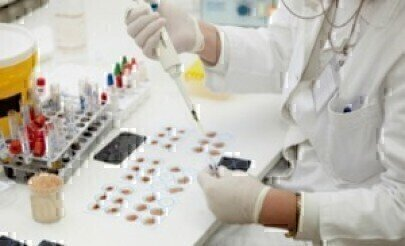GC, MDGC

•What is a Response Factor?

Aug 08 2014 Read 193446 Times

In chromatography, a response factor is defined as the ratio between the concentration of a compound being analysed and the response of the detector to that compound. A chromatogram will show a response from a detector as a peak. While there are several ways to quantify the peak, one of the most common is peak area, thus:

Response Factor = Peak Area / Concentration

It is important to remember that variations in a gas chromatography (GC) system and analysis methodology can be the cause of a deviation in the response factor.

Quantitative Analysis

Quantitative analysis is the determination of a compound’s concentration in a sample (analyte). Response factors are important when using GC for quantitative analysis. Therefore, for reproducibility in sample measurements, a method of eliminating variability in the response factor must be used. One of the easiest ways to eliminate variation in the response factor is to use relative response factors and an internal standard to calibrate the GC.

Relative Response Factors (RRFs)

The response factors calculated for each analyte are then used to establish the RRF between the two analytes thus:

Relative Response Factor (RRF) = Response Factor A / Response Factor B

The RRF can be used to calculate the unknown concentration of analyte A in the presence of a known concentration of analyte B using the following equation:

Concentration A = Peak area A / Peak area B   x   1 / RRF   x   Concentration B

Using RRFs is beneficial when one of the samples is an internal standard - a calibration then allows multiple analyses to be run on different samples. A discussion of how RRFs are used in pharmaceutical R&D involving GC can be found in Rome and McIntyre (2013).

Internal Standard

An internal standard (IS) is a compound that is similar in physical and chemical characteristics to the sample being analysed. It must be inert to the sample and must not react with the sample or any solvent used to dilute or prepare it for GC.

Steps in a Quantitative Analysis

To determine the ratio of two compounds A and B in a sample, first the GC has to be calibrated. Once the calibration is complete, the sample can be analysed and the concentrations of A and B in the unknown sample determined. A simplified procedure is:

1) Prepare a calibration sample, which should contain compounds A and B at a known concentration. Add a known amount of IS. The same concentration of IS should be used in the calibration and analysis samples.

2) Introduce the calibration sample into the GC. Since the concentrations of A, B and IS are known, the results can be used to calculate the RRFs of A and B with respect to the IS thus:

RRFB = Response factor B / Response factor IS

3) Make up a sample of the unknown and a known concentration of IS and inject into the GC. After analysis, the results required are the peak areas of A, B and IS.

4) A simple calculation computes the concentration of A and B in the unknown.

a. Let the RRFA = 1.13 from step 2

b. Then using the Response Factor and RRF equations:

RRFA=  (Peak Area A / Conc. A) / (Peak Area IS / Conc. IS)

c. The concentration of A can be determined  by rearrangement of this equation:

Conc. A = Peak Area A x (Conc. IS / Peak Area IS) x (1 / 1.13)

d. Since we know the peak areas of A and IS (from step 3), and the concentration of IS used, we can calculate the concentration of A.

e. A similar calculation yields the concentration of B.

References

Rome, K. & McIntyre, A. (2012). Intelligent use of Relative Response Factors in Gas Chromatography-Flame Ionisation Detection. Chromatography Today, 52.

Do you like or dislike what you have read? Why not post a comment to tell others / the manufacturer and our Editor what you think. To leave comments please complete the form below. Providing the content is approved, your comment will be on screen in less than 24 hours. Leaving comments on product information and articles can assist with future editorial and article content. Post questions, thoughts or simply whether you like the content.

What Does Dark Chocolate Actually Smell Like? - Chromatography Sniffs It...

Jun 14 2019 Read 1198 Times

Using the Agilent J&W DB-HeaveyWAX columns as the second dimenstion in c...

Mar 27 2019 Read 1356 Times

How is Chromatography Changing Fire Debris Analysis?

Mar 13 2019 Read 1279 Times

What Do Roses Smell Of? — Chromatography Investigates

Jul 10 2018 Read 3374 Times

Does Gastric Bypass Surgery Affect Insulin Sens...

Chromatography Today - September 2019

September 2019

In This Edition Fundamental ASpects The Quest for True Ultra High Performance Supercritical Fluid Chromatography Walk-up SFC-MS for Fast Purification of reaction Mixtures within Discover...

Walk-up SFC-MS for Fast Purification of reactio...

Events

MicroTAS 2019

Oct 27 2019 Basel, Switzerland

Eastern Analytical Symposium

Nov 18 2019 Princeton, NJ, USA

HTC-16

Jan 29 2020 Ghent, Belgium

MEDLAB MIDDLE EAST

Feb 03 2020 Dubai, UAE

China Lab 2020

Mar 11 2020 Guangzhou, China